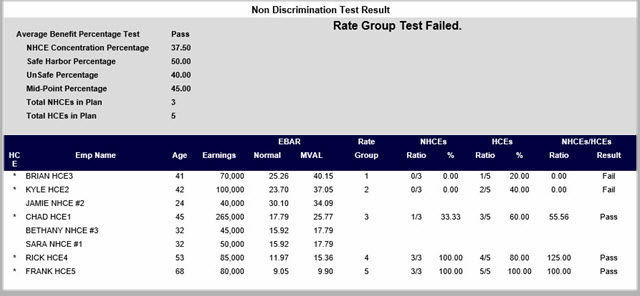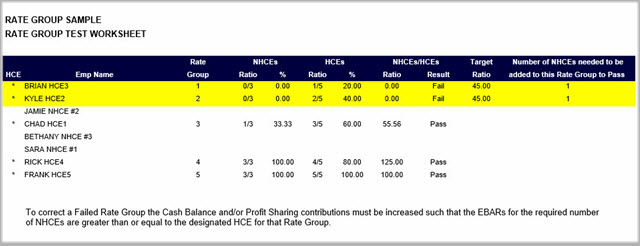Rate Group Test Worksheet Report

 Normal and MVAL EBARS The normal accrual rate for an employee for the plan year is the increase in the employee’s accrued benefit (within the meaning of section 411(a)(7)(A)(i) during the measurement period.   The most valuable optional form of benefit used to determine the most valuable accrual rate reflects the value of all Optional Forms of Benefits accrued or treated as accrued that are payable in any form and at any time under the plan.   What is a Rate Group? A Rate Group consists of one HCE and all other employees whose Normal and MVAL EBARS are equal to or greater than the EBARS of the HCE.   In order to determine whether a plan satisfies the general test, the plan is broken down into rate groups, or “mini plans”. Each HCE who receives an allocation or an accrual rate forms a rate group. Every other participant who has an equal or greater allocation rate or accrual rate than the HCE is a member of that rate group.   In our example, the first Rate group consists of Brian HCE3 only because there are no other employees with a Normal and MVAL EBARS greater than Brian.   Rate Group 2 consists of Kyle HCE2 and Brian HCE3.  That’s because Brian’s Normal and MVAL EBARS are greater than Kyle.   Rate Group 3 consists of Chad HCE1 and Jamie, Kyle and Brian.   To simplify things, this report, is sorted by both Normal and MVAL EBARS in descending order.   Now, it should be easy to see that Rate Group 4 consists of Rick HCE4 and all the employees above Rate Group 4.   Finally, Rate Group 5 consists of Frank HCE5 and all the other employees.   What is a Ratio Percentage? A Ratio Percentage must be calculated for each Rate Group. This calculation is done in three steps:   Step 1: Count the number of NHCE's in each Rate Group and divide this by the total NHCE's in the Plan.   Step 2: Count the number of HCE's in the Rate Group and divide this by the total HCE's in the Plan.   Step 3: The Ratio Percentage for a Rate Group is Step 1 divided by Step 2.     Look at Rate Group 3 in the example below: The Ratio Percentage of Rate Group 3 is 1/3 (one out of 3 NHCE's) divided by 3/5 (3 HCE's out of 5 HCE's) equals 55.56%.If the Ratio Percentage for all Rate Groups is 70% or more, the plan automatically passes the Rate Group test.   In this example, our Ratio Percentages are all lower than 70%.   However, if the Plan passes the Average Benefit Percentage Test, the Ratio Percentages must only be greater than the Mid-Point Percentage of 45% to pass.   But since Rate Group 1 and Rate Group 2 are both zero, we fail.   The worksheet below, shows that we only need 1 NHCE to have Normal & MVAL EBAR greater than Brian & Kyle’s EBARS to pass. You should then go to the Classes and increase the Profit sharing for the NHCE's until it passes the Rate Group Test.